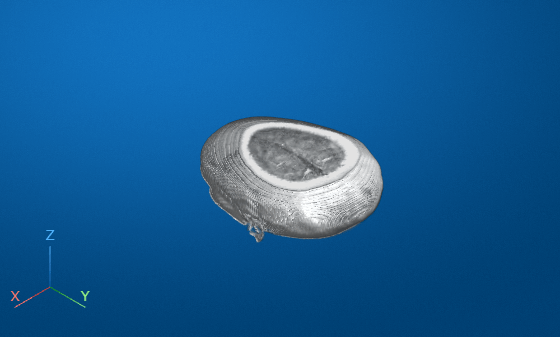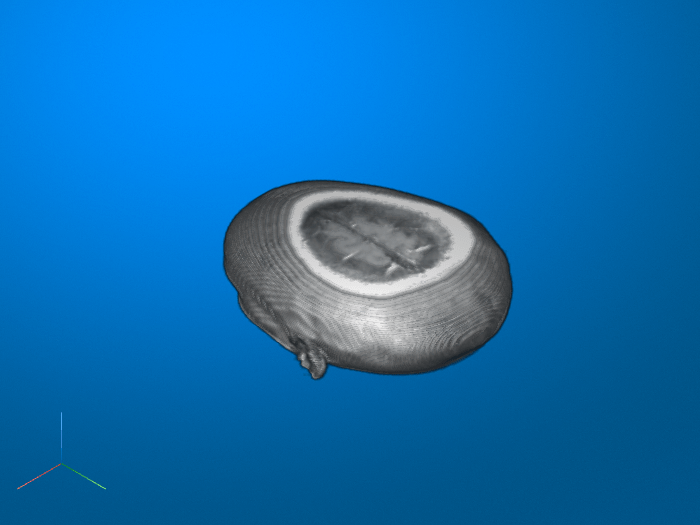# randomCropWindow3d

Create randomized cuboidal cropping window

## Syntax

``win = randomCropWindow3d(inputSize,targetSize)``

## Description

example

````win = randomCropWindow3d(inputSize,targetSize)` determines the window to crop from a 3-D input image of size `inputSize` such that the size of the cropped image is `targetSize`. The coordinates of the window are selected from a random position in the input image.```

## Examples

collapse all

Read a 3-D MRI volume. Use the `squeeze` function to remove any singleton dimensions.

```load mri; D = squeeze(D);```

Display the volume.

`volshow(D);`Specify the target size of the cropping window.

`targetSize = [64 64 10];`

Create a random crop window that crops the input volume from a randomly-selected position.

`win = randomCropWindow3d(size(D),targetSize);`

Crop the volume using the random crop window.

`Dcrop = imcrop3(D,win);`

Display the cropped volume.

`volshow(Dcrop);`## Input Arguments

collapse all

Input image size, specified as one of the following.

Type of Input ImageFormat of `inputSize`
3-D grayscale or binary image3-element vector of positive integers of the form ```[height width depth]```
3-D RGB or multispectral image4-element vector of positive integers of the form ```[height width depth channels]```

Data Types: `single` | `double` | `int8` | `int16` | `int32` | `int64` | `uint8` | `uint16` | `uint32` | `uint64`

Target image size, specified as one of the following.

Type of Target ImageFormat of `targetSize`
3-D grayscale or binary image3-element vector of positive integers of the form ```[height width depth]```
3-D RGB or multispectral image4-element vector of positive integers of the form ```[height width depth channels]```

Data Types: `single` | `double` | `int8` | `int16` | `int32` | `int64` | `uint8` | `uint16` | `uint32` | `uint64`

## Output Arguments

collapse all

Cropping window, returned as a `Cuboid` object.

## Version History

Introduced in R2019b